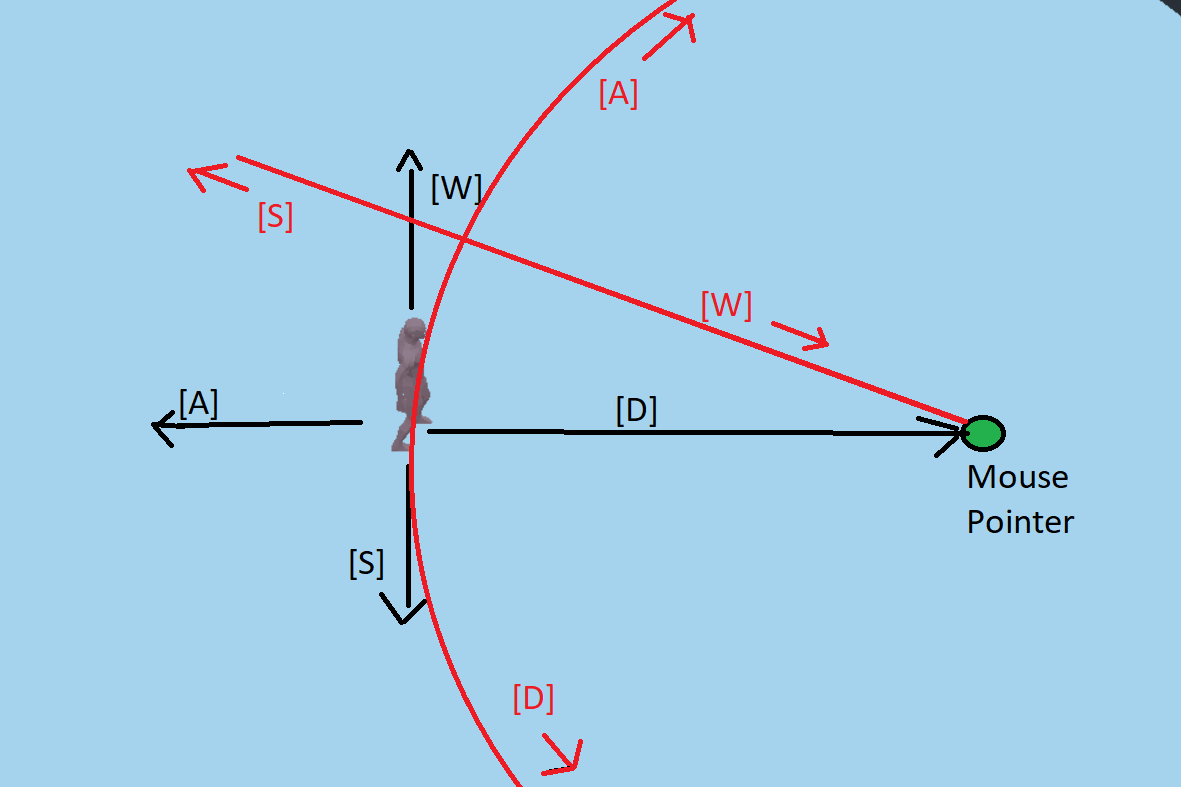Hi.
I am struggling with the movement i my game. What i am looking for is a system in wich the player is always facing the mouse pointer and where the movement is relative to the pointer aswell. So that [W]/forward wil mean that the player moves towards the pointer and the sideways keys will lead to the player strafing in a circle around the pointer.
I have tried to illustrate it in the picture below. The black illustrates the current system and the red is the one i would like to make.Below is the current code for the character movement:

``````extends KinematicBody

var velocity = Vector3.ZERO
var pos
var basis = Basis()
var jump = false
var jump_speed = 10

export var gravity = Vector3.DOWN * 10

var rayOrigin = Vector3()
var rayEnd = Vector3()

const SPEED = 25
const SPRINT_SPEED = 35

\$AnimationPlayer.play("Idle")

func _physics_process(delta):
velocity += gravity * delta
look_at_mouse_point()
get_input()

velocity = move_and_slide(velocity, Vector3.UP)

if jump and is_on_floor():
velocity.y = jump_speed

func get_input():

var vy = velocity.y
velocity = Vector3.ZERO

if Input.is_action_pressed("right"):
\$AnimationPlayer.play("Run")
velocity += transform.basis.x * SPEED
if Input.is_action_pressed("left"):
\$AnimationPlayer.play("Run")
velocity += transform.basis.x * -SPEED
if Input.is_action_pressed("forward"):
\$AnimationPlayer.play("Run")
velocity += transform.basis.z * -SPEED
if Input.is_action_pressed("backwards"):
\$AnimationPlayer.play("Run")
velocity += transform.basis.z * SPEED

jump = false
if Input.is_action_just_pressed("jump"):
jump = true

if velocity.x == 0 and velocity.z == 0 :
\$AnimationPlayer.play("Idle")

velocity.y = vy

func look_at_mouse_point():

var space_state = get_world().direct_space_state
var mouse_position = get_viewport().get_mouse_position()
rayOrigin = camera.project_ray_origin(mouse_position)
rayEnd = rayOrigin + camera.project_ray_normal(mouse_position) *2000

var intersection = space_state.intersect_ray(rayOrigin, rayEnd)

if not intersection.empty():
pos = intersection.position
\$Armature.look_at(Vector3(pos.x, translation.y, pos.z), Vector3.UP)
``````

EDIT: Fixed picture and Codeblocks wich was not showing correctly

Godot version 3.4.2
in Engine
edited

I have now solved the problem so i'll just write my solution if anyone else should need the same system as I. It turned out the solution was not that complicated.

``````    if Input.is_action_pressed("right"):
\$AnimationPlayer.play("Run")
velocity += \$Armature.transform.basis.x * SPEED
if Input.is_action_pressed("left"):
\$AnimationPlayer.play("Run")
velocity += \$Armature.transform.basis.x * -SPEED
if Input.is_action_pressed("forward"):
\$AnimationPlayer.play("Run")
velocity += \$Armature.transform.basis.z * -SPEED
if Input.is_action_pressed("backwards"):
\$AnimationPlayer.play("Run")
velocity += \$Armature.transform.basis.z * SPEED
``````

I have added \$Armature before transforming the basis. This seems locical looking back at it as i want the transform to happen local to the character object. (Armature in this context is a spatial within the Caharacter object containing the skeleton and mesh)

This gave me another problem, the mouse would move when the character did. This was solved by moving the logic of `look_at_mouse_point()` to a script in the level instead of the characterscript. This is how the level script turned out.

``````extends StaticBody

var pos
var rayOrigin = Vector3()
var rayEnd = Vector3()

func _physics_process(delta):
var space_state = get_world().direct_space_state
var mouse_position = get_viewport().get_mouse_position()
rayOrigin = camera.project_ray_origin(mouse_position)
rayEnd = rayOrigin + camera.project_ray_normal(mouse_position) *2000

var intersection = space_state.intersect_ray(rayOrigin, rayEnd)

if not intersection.empty():
pos = intersection.position
\$Character/Armature.look_at(Vector3(pos.x, \$Character.translation.y,
pos.z), Vector3.UP)
``````

+1 vote

You're absolutely on the right lines with:

``````func look_at_mouse_point():
var space_state = get_world().direct_space_state
var mouse_position = get_viewport().get_mouse_position()
rayOrigin = camera.project_ray_origin(mouse_position)
rayEnd = rayOrigin + camera.project_ray_normal(mouse_position) *2000
var intersection = space_state.intersect_ray(rayOrigin, rayEnd)

if not intersection.empty():
pos = intersection.position
\$Armature.look_at(Vector3(pos.x, translation.y, pos.z), Vector3.UP)
``````

That's solid code. Maybe change `pos = intersection.position` to `pos = intersection.collider.global_transform.origin` to point at the origin of the object rather than where the mouse hit it (if that's what you want to happen). Also, if there's anything in the way that you want ignored you can add it to an array like this: `space_state.intersect_ray(cam_origin, cam_end, [obj1, obj2])`

In what way is this not giving you the result you want?

by (2,156 points)